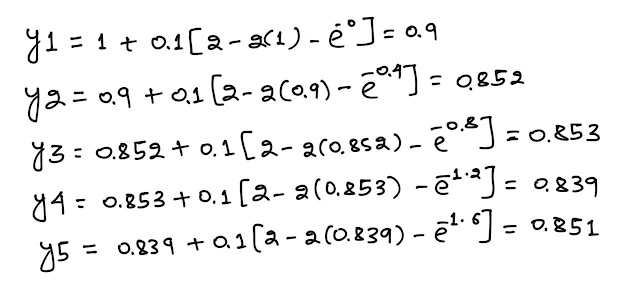To solve the differential equation using Euler's Method with given initial value conditions y(0)=1, step size h=0.1

Given DE is 👇

A PC/laptop.
Scilab 6.0.0 must be installed.

THEORY:
Euler's method is a method of approximation to solve first order DE with a given initial value problem that is t(0)=0 & y(0)=1.

The differential equation is

Then by Euler Method we can calculate
t(i+1)=t(i)+h
And     y(i+1)=y(i)+h*f(t(i),y(i))
``
CODING:
```clc
function dy=f(t, y)          //defining the function
dy=2-2*y-exp(-4*t);        //given DE
endfunction
t0=0;                       //initial value of t
y0=1;
tf=0.5;
h=0.1;
n=(tf-t0)/h;
t(1)=t0;
y(1)=y0;

for i=1:n+1
t(i+1)=t(i)+h;              //Euler's Method
y(i+1)=y(i)+h*f(t(i),y(i))
z=[i,t(i+1),y(i+1)]
disp(z);
end
clf```
OUTPUT: